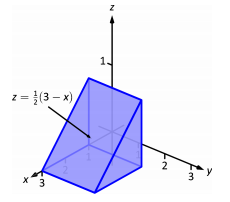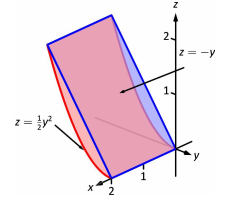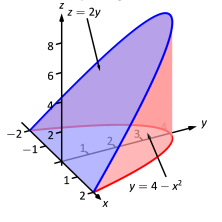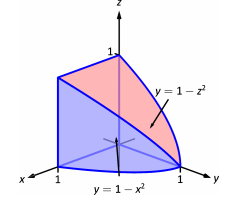# Triple Integrals (Exercises 2)

$$\newcommand{\vecs}{\overset { \rightharpoonup} {\mathbf{#1}} }$$ $$\newcommand{\vecd}{\overset{-\!-\!\rightharpoonup}{\vphantom{a}\smash {#1}}}$$$$\newcommand{\id}{\mathrm{id}}$$ $$\newcommand{\Span}{\mathrm{span}}$$ $$\newcommand{\kernel}{\mathrm{null}\,}$$ $$\newcommand{\range}{\mathrm{range}\,}$$ $$\newcommand{\RealPart}{\mathrm{Re}}$$ $$\newcommand{\ImaginaryPart}{\mathrm{Im}}$$ $$\newcommand{\Argument}{\mathrm{Arg}}$$ $$\newcommand{\norm}{\| #1 \|}$$ $$\newcommand{\inner}{\langle #1, #2 \rangle}$$ $$\newcommand{\Span}{\mathrm{span}}$$ $$\newcommand{\id}{\mathrm{id}}$$ $$\newcommand{\Span}{\mathrm{span}}$$ $$\newcommand{\kernel}{\mathrm{null}\,}$$ $$\newcommand{\range}{\mathrm{range}\,}$$ $$\newcommand{\RealPart}{\mathrm{Re}}$$ $$\newcommand{\ImaginaryPart}{\mathrm{Im}}$$ $$\newcommand{\Argument}{\mathrm{Arg}}$$ $$\newcommand{\norm}{\| #1 \|}$$ $$\newcommand{\inner}{\langle #1, #2 \rangle}$$ $$\newcommand{\Span}{\mathrm{span}}$$$$\newcommand{\AA}{\unicode[.8,0]{x212B}}$$

## Terms and Concepts

1. The strategy for establishing bounds for triple integrals is "from ________ to ________, then from ________ to ________ and then from ________ to ________."

We integrate from surface to surface, then from curve to curve and then from point to point.

2. Give an informal interpretation of what $$\int\int\int_Q \,dV$$ means.

$$\int\int\int_Q \,dV$$ = Volume of the solid region $$Q$$

3. Give two uses of triple integration.

To compute total mass or average density of a solid object, given a density function or to compute the average temperature in a solid region or object.

4. If an object has a constant density $$\delta$$ and a volume $$V$$, what is its mass?

It's mass is $$\delta V$$.

## Volume of Solid Regions

In Exercises 5-8, two surfaces $$f_1(x,y)$$ and $$f_2(x,y)$$ and a region $$R$$ in the $$xy$$-plane are given. Set up and evaluate the triple integral that represents the volume between these surfaces over $$R$$.

5. $$f_1(x,y) = 8-x^2-y^2,\,f_2(x,y) =2x+y;$$
$$R$$ is the square with corners $$(-1,-1)$$ and $$(1,1)$$.

6. $$f_1(x,y) = x^2+y^2,\,f_2(x,y) =-x^2-y^2;$$
$$R$$ is the square with corners $$(0,0)$$ and $$(2,3)$$.

7. $$f_1(x,y) = \sin x \cos y,\,f_2(x,y) =\cos x \sin y +2;$$
$$R$$ is the triangle with corners $$(0,0), \,(\pi , 0)$$ and $$(\pi,\pi)$$.

8. $$f_1(x,y) = 2x^2+2y^2+3,\,f_2(x,y) =6-x^2-y^2;$$
$$R$$ is the circle $$x^2+y^2=1$$.

In Exercises 9-16, a domain $$D$$ is described by its bounding surfaces, along with a graph. Set up the triple integral that gives the volume of $$D$$ in the indicated order of integration, and evaluate the triple integral to find this volume.

9. $$D$$ is bounded by the coordinate planes and $$z=2-\frac{2}{3}x-2y$$.
Evaluate the triple integral with order $$dz\,dy\,dx$$.10. $$D$$ is bounded by the planes $$y=0,y=2,x=1,z=0$$ and $$z=(2-x)/2$$.
Evaluate the triple integral with order $$dx\,dy\,dz$$.11. $$D$$ is bounded by the planes $$x=0,x=2,z=-y$$ and by $$z=y^2/2$$.
Evaluate the triple integral with order $$dy\,dz\,dx$$.12. $$D$$ is bounded by the planes $$z=0,y=9, x=0$$ and by $$z=\sqrt{y^2-9x^2}$$.
Do not evaluate any triple integral. Just set this one up.13. $$D$$ is bounded by the planes $$x=2,y=1,z=0$$ and $$z=2x+4y-4$$.
Evaluate the triple integral with order $$dx\,dy\,dz$$.14. $$D$$ is bounded by the plane $$z=2y$$ and by $$y=4-x^2$$.
Evaluate the triple integral with order $$dz\,dy\,dx$$.15. $$D$$ is bounded by the coordinate planes and $$y=1-x^2$$ and $$y=1-z^2$$.
Do not evaluate any triple integral. Which order would be easier to evaluate: $$dz\,dy\,dx$$ or $$dy\,dz\,dx$$? Explain why.16. $$D$$ is bounded by the coordinate planes and by $$z=1-y/3$$ and $$z=1-x$$.
Evaluate the triple integral with order $$dx\,dy\,dz$$.In Exercises 17-20, evaluate the triple integral.

17. $$\displaystyle \int_{-\pi/2}^{\pi/2}\int_{0}^{\pi}\int_{0}^{\pi} (\cos x \sin y \sin z )\,dz\,dy\,dx$$

18. $$\displaystyle \int_{0}^{1}\int_{0}^{x}\int_{0}^{x+y} (x+y+z )\,dz\,dy\,dx$$

19. $$\displaystyle \int_{0}^{\pi}\int_{0}^{1}\int_{0}^{z} (\sin (yz))\,dx\,dy\,dz$$

20. $$\displaystyle \int_{\pi}^{\pi^2}\int_{x}^{x^3}\int_{-y^2}^{y^2} (\cos x \sin y \sin z )\,dz\,dy\,dx$$

In the following exercises, evaluate the triple integrals over the rectangular solid box $$B$$.

$\iiint_B (2x + 3y^2 + 4z^3) \space dV,$ where $$B = \{(x,y,z) | 0 \leq x \leq 1, \space 0 \leq y \leq 2, \space 0 \leq z \leq 3\}$$

[Hide Solution]

$$192$$

$\iiint_B (xy + yz + xz) \space dV,$ where $$B = \{(x,y,z) | 1 \leq x \leq 2, \space 0 \leq y \leq 2, \space 1 \leq z \leq 3\}$$

$\iiint_B (x \space cos \space y + z) \space dV,$ where $$B = \{(x,y,z) | 0 \leq x \leq 1, \space 0 \leq y \leq \pi, \space -1 \leq z \leq 1\}$$

[Hide solution]

$$0$$

$\iiint_B (z \space sin \space x + y^2) \space dV,$ where $$B = \{(x,y,z) | 0 \leq x \leq \pi, \space 0 \leq y \leq 1, \space -1 \leq z \leq 2\}$$

In the following exercises, change the order of integration by integrating first with respect to $$z$$, then $$x$$, then $$y$$.

$\int_0^1 \int_1^2 \int_2^3 (x^2 + ln \space y + z) \space dx \space dy \space dz$

[Hide Solution]

$\int_0^1 \int_1^2 \int_2^3 (x^2 + ln \space y + z) \space dx \space dy \space dz = \frac{35}{6} + 2 \space ln 2$

$\int_0^1 \int_{-1}^1 \int_0^3 (ze^x + 2y) \space dx \space dy \space dz$

$\int_{-1}^2 \int_1^3 \int_0^4 \left(x^2z + \frac{1}{y}\right) \space dx \space dy \space dz$

[Hide solution]

$\int_{-1}^2 \int_1^3 \int_0^4 \left(x^2z + \frac{1}{y}\right) \space dx \space dy \space dz = 64 + 12 \space ln \space 3$

$\int_1^2 \int_{-2}^{-1} \int_0^1 \frac{x + y}{z} \space dx \space dy \space dz$

Let $$F$$, $$G$$, and $$H$$ be continuous functions on $$[a,b]$$, $$[c,d]$$, and $$[e,f]$$, respectively, where $$a, \space b, \space c, \space d, \space e$$, and $$f$$ are real numbers such that $$a < b, \space c < d$$, and $$e < f$$. Show that

$\int_a^b \int_c^d \int_e^f F (x) \space G (y) \space H(z) \space dz \space dy \space dx = \left(\int_a^b F(x) \space dx \right) \left(\int_c^d G(y) \space dy \right) \left(\int_e^f H(z) \space dz \right).$

Let $$F$$, $$G$$, and $$H$$ be differential functions on $$[a,b]$$, $$[c,d]$$, and $$[e,f]$$, respectively, where $$a, \space b, \space c, \space d, \space e$$, and $$f$$ are real numbers such that $$a < b, \space c < d$$, and $$e < f$$. Show that

$\int_a^b \int_c^d \int_e^f F' (x) \space G' (y) \space H'(z) \space dz \space dy \space dx = [F (b) - F (a)] \space [G(d) - G(c)] \space H(f) - H(e)].$

In the following exercises, evaluate the triple integrals over the bounded region

$$E = \{(x,y,z) | a \leq x \leq b, \space h_1 (x) \leq y \leq h_2 (x), \space e \leq z \leq f \}.$$

$\iiint_E (2x + 5y + 7z) \space dV,$ where $$E = \{(x,y,z) | 0 \leq x \leq 1, \space 0 \leq y \leq -x + 1, \space 1 \leq z \leq 2\}$$

[Hide solution]

$$\frac{77}{12}$$

$\iiint_E (y \space ln \space x + z) \space dV,$ where $$E = \{(x,y,z) | 1 \leq x \leq e, \space 0 \leq y ln \space x, \space 0 \leq z \leq 1\}$$

$\iiint_E (sin \space x + sin \space y) dV,$ where $$E = \{(x,y,z) | 0 \leq x \leq \frac{\pi}{2}, \space -cos \space x \leq y cos \space x, \space -1 \leq z \leq 1 \}$$

[Hide Solution]

$$2$$

$\iiint_E (xy + yz + xz ) dV$ where $$E = \{(x,y,z) | 0 \leq x \leq 1, \space -x^2 \leq y \leq x^2, \space 0 \leq z \leq 1 \}$$

In the following exercises, evaluate the triple integrals over the indicated bounded region $$E$$.

$\iiint_E (x + 2yz) \space dV,$ where $$E = \{(x,y,z) | 0 \leq x \leq 1, \space 0 \leq y \leq x, \space 0 \leq z \leq 5 - x - y \}$$

[Hide Solution]

$$\frac{430}{120}$$

$\iiint_E (x^3 + y^3 + z^3) \space dV,$ where $$E = \{(x,y,z) | 0 \leq x \leq 2, \space 0 \leq y \leq 2x, \space 0 \leq z \leq 4 - x - y \}$$

$\iiint_E y \space dV,$ where $$E = \{(x,y,z) | -1 \leq x \leq 1, \space -\sqrt{1 - x^2} \leq y \leq \sqrt{1 - x^2}, \space 0 \leq z \leq 1 - x^2 - y^2 \}$$

[Hide Solution]

$$0$$

$\iiint_E x \space dV,$ where $$E = \{(x,y,z) | -2 \leq x \leq 2, \space -4\sqrt{1 - x^2} \leq y \leq \sqrt{4 - x^2}, \space 0 \leq z \leq 4 - x^2 - y^2 \}$$

In the following exercises, evaluate the triple integrals over the bounded region $$E$$ of the form

$$E = \{(x,y,z) | g_1 (y) \leq x \leq g_2(y), \space c \leq y \leq d, \space e \leq z \leq f \}$$.

$\iiint_E x^2 \space dV,$ where $$E = \{(x,y,z) | 1 - y^2 \leq x \leq y^2 - 1, \space -1 \leq y \leq 1, \space 1 \leq z \leq 2 \}$$

[Hide Solution]

$$-\frac{64}{105}$$

$\iiint_E (sin \space x + y) \space dV,$ where $$E = \{(x,y,z) | -y^4 \leq x \leq y^4, \space 0 \leq y \leq 2, \space 0 \leq z \leq 4\}$$

$\iiint_E (x - yz) \space dV,$ where $$E = \{(x,y,z) | -y^6 \leq x \leq \sqrt{y}, \space 0 \leq y \leq 1x, \space -1 \leq z \leq 1 \}$$

[Hide Solution]

$$\frac{11}{26}$$

$\iiint_E z \space dV,$ where $$E = \{(x,y,z) | 2 - 2y \leq x \leq 2 + \sqrt{y}, \space 0 \leq y \leq 1x, \space 2 \leq z \leq 3 \}$$

In the following exercises, evaluate the triple integrals over the bounded region

$$E = \{(x,y,z) | g_1(y) \leq x \leq g_2(y), \space c \leq y \leq d, \space u_1(x,y) \leq z \leq u_2 (x,y) \}$$

$\iiint_E z \space dV,$ where $$E = \{(x,y,z) | -y \leq x \leq y, \space 0 \leq y \leq 1, \space 0 \leq z \leq 1 - x^4 - y^4 \}$$

[Hide Solution]

$$\frac{113}{450}$$

$\iiint_E (xz + 1) \space dV,$ where $$E = \{(x,y,z) | 0 \leq x \leq \sqrt{y}, \space 0 \leq y \leq 2, \space 0 \leq z \leq 1 - x^2 - y^2 \}$$

$\iiint_E (x - z) \space dV,$ where $$E = \{(x,y,z) | - \sqrt{1 - y^2} \leq x \leq y, \space 0 \leq y \leq \frac{1}{2}x, \space 0 \leq z \leq 1 - x^2 - y^2 \}$$

[Hide Solution]

$$\frac{1}{160}(6 \sqrt{3} - 41)$$

$\iiint_E (x + y) \space dV,$ where $$E = \{(x,y,z) | 0 \leq x \leq \sqrt{1 - y^2}, \space 0 \leq y \leq 1x, \space 0 \leq z \leq 1 - x \}$$

In the following exercises, evaluate the triple integrals over the bounded region

$$E = \{(x,y,z) | (x,y) \in D, \space u_1 (x,y) x \leq z \leq u_2 (x,y) \}$$, where $$D$$ is the projection of $$E$$ onto the $$xy$$-plane

$\iint_D \left(\int_1^2 (x + y) \space dz \right) \space dA,$ where $$D = \{(x,y) | x^2 + y^2 \leq 1\}$$

[Hide Solution]

$$\frac{3\pi}{2}$$

$\iint_D \left(\int_1^3 x (z + 1)\space dz \right) \space dA,$ where $$D = \{(x,y) | x^2 -y^2 \geq 1, \space x \leq \sqrt{5}\}$$

$\iint_D \left(\int_0^{10-x-y} (x + 2z) \space dz \right) \space dA,$ where $$D = \{(x,y) | y \geq 0, \space x \geq 0, \space x + y \leq 10\}$$

[Hide Solution]

$$1250$$

$\iint_D \left(\int_0^{4x^2+4y^2} y \space dz \right) \space dA,$ where $$D = \{(x,y) | x^2 + y^2 \leq 4, \space y \geq 1, \space x \geq 0\}$$

The solid $$E$$ bounded by $$y^2 + z^2 = 9, \space z = 0$$, and $$x = 5$$ is shown in the following figure. Evaluate the integral $\iiint_E z \space dV$ by integrating first with respect to $$z$$, then $$y$$, and then $$x$$.[Hide Solution]

$\int_0^5 \int_{-3}^3 \int_0^{\sqrt{9-y^2}} z \space dz \space dy \space dx = 90$

The solid $$E$$ bounded by $$y = \sqrt{x}, \space x = 4, \space y = 0$$, and $$z = 1$$ is given in the following figure. Evaluate the integral $\iiint_E xyz \space dV$ by integrating first with respect to $$x$$, then $$y$$, and then $$z$$.[T] The volume of a solid $$E$$ is given by the integral $\int_{-2}^0 \int_x^0 \int_0^{x^2+y^2} dz \space dy \space dx.$ Use a computer algebra system (CAS) to graph $$E$$ and find its volume. Round your answer to two decimal places.

[Hide Solution]

$$V = 5.33$$[T] The volume of a solid $$E$$ is given by the integral $\int_{-1}^0 \int_{-x^3}^0 \int_0^{1+\sqrt{x^2+y^2}} dz \space dy \space dx.$ Use a CAS to graph $$E$$ and find its volume $$V$$. Round your answer to two decimal places.

In the following exercises, use two circular permutations of the variables $$x, \space y,$$ and $$z$$ to write new integrals whose values equal the value of the original integral. A circular permutation of $$x, \space y$$, and $$z$$ is the arrangement of the numbers in one of the following orders: $$y, \space z,$$ and $$x$$ or $$z, \space x,$$ and $$y$$.

$\int_0^1 \int_1^3 \int_2^4 (x^2z^2 + 1) dx \space dy \space dz$

[Hide Solution]

$\int_0^1 \int_1^3 \int_2^4 (y^2z^2 + 1) dz \space dx \space dy;$ $\int_0^1 \int_1^3 \int_2^4 (x^2z^2 + 1) dx \space dy \space dz$

$\int_0^3 \int_0^1 \int_0^{-x+1} (2x + 5y + 7z) dy \space dx \space dz$

$\int_0^1 \int_{-y}^y \int_0^{1-x^4-y^4} ln \space x dz \space dx \space dy$

$\int_{-1}^1 \int_0^1 \int_{-y^6}^{\sqrt{y}} (x + yz) dx \space dy \space dz$

Set up the integral that gives the volume of the solid $$E$$ bounded by $$y^2 = x^2 + z^2$$ and $$y = a^2$$, where $$a > 0$$.

[Hide Solution]

$V = \int_{-a}^a \int_{-\sqrt{a^2-z^2}}^{\sqrt{a^2-z^2}} \int_{\sqrt{x^2+z^2}}^{a^2} dy \space dx \space dz$

Set up the integral that gives the volume of the solid $$E$$ bounded by $$x = y^2 + z^2$$ and $$x = a^2$$, where $$a > 0$$.

Find the average value of the function $$f(x,y,z) = x + y + z$$ over the parallelepiped determined by $$x + 0, \space x = 1, \space y = 0, \space y = 3, \space z = 0$$, and $$z = 5$$.

[Hide Solution]

$$\frac{9}{2}$$

Find the average value of the function $$f(x,y,z) = xyz$$ over the solid $$E = [0,1] \times [0,1] \times [0,1]$$ situated in the first octant.

Find the volume of the solid $$E$$ that lies under the plane $$x + y + z = 9$$ and whose projection onto the $$xy$$-plane is bounded by $$x = sin^{-1} y, \space y = 0$$, and $$x = \frac{\pi}{2}$$.

Consider the pyramid with the base in the $$xy$$-plane of $$[-2,2] \times [-2,2]$$ and the vertex at the point $$(0,0,8)$$.

a. Show that the equations of the planes of the lateral faces of the pyramid are $$4y + z = 8, \space 4y - z = -8, \space 4x + z = 8$$, and $$-4x + z = 8$$.

b. Find the volume of the pyramid.

[Hide Solution]

a. Answers may vary; b. $$\frac{128}{3}$$

Consider the pyramid with the base in the $$xy$$-plane of $$[-3,3] \times [-3,3]$$ and the vertex at the point $$(0,0,9)$$.

a. Show that the equations of the planes of the side faces of the pyramid are $$3y + z = 9, \space 3y + z = 9, \space y = 0$$ and $$x = 0$$.

b. Find the volume of the pyramid.

The solid $$E$$ bounded by the sphere of equation $$x^2 + y^2 + z^2 = r^2$$ with $$r > 0$$ and located in the first octant is represented in the following figure.a. Write the triple integral that gives the volume of $$E$$ by integrating first with respect to $$z$$, then with $$y$$, and then with $$x$$.

b. Rewrite the integral in part a. as an equivalent integral in five other orders.

[Hide Solution]

$a. \space \int_0^4 \int_0^{\sqrt{r^2-x^2}} \int_0^{\sqrt{r^2-x^2-y^2}} dz \space dy \space dx; \space b. \space \int_0^2 \int_0^{\sqrt{r^2-x^2}} \int_0^{\sqrt{r^2-x^2-y^2}} dz \space dx \space dy,$

$\int_0^r \int_0^{\sqrt{r^2-x^2}} \int_0^{\sqrt{r^2-x^2-y^2}} dy \space dx \space dz, \space \int_0^r \int_0^{\sqrt{r^2-x^2}} \int_0^{\sqrt{r^2-x^2-y^2}} dy \space dz \space dx,$

$\int_0^r \int_0^{\sqrt{r^2-x^2}} \int_0^{\sqrt{r^2-x^2-y^2}} dx \space dy \space dz, \space \int_0^r \int_0^{\sqrt{r^2-x^2}} \int_0^{\sqrt{r^2-x^2-y^2}} dx \space dz \space dy,$

The solid $$E$$ bounded by the sphere of equation $$9x^2 + 4y^2 + z^2 = 1$$ and located in the first octant is represented in the following figure.a. Write the triple integral that gives the volume of $$E$$ by integrating first with respect to $$z$$ then with $$y$$ and then with $$x$$.

b. Rewrite the integral in part a. as an equivalent integral in five other orders.

Find the volume of the prism with vertices $$(0,0,0), \space (2,0,0), \space (2,3,0), \space (0,3,0), \space (0,0,1)$$, and $$(2,0,1)$$.

[Hide Solution]

$$3$$

Find the volume of the prism with vertices $$(0,0,0), \space (4,0,0), \space (4,6,0), \space (0,6,0), \space (0,0,1)$$, and $$(4,0,1)$$.

The solid $$E$$ bounded by $$z = 10 - 2x - y$$ and situated in the first octant is given in the following figure. Find the volume of the solid.[Hide Solution]

$$\frac{250}{3}$$

The solid $$E$$ bounded by $$z = 1 - x^2$$ and situated in the first octant is given in the following figure. Find the volume of the solid.The midpoint rule for the triple integral $\iiint_B f(x,y,z) dV$ over the rectangular solid box $$B$$ is a generalization of the midpoint rule for double integrals. The region $$B$$ is divided into subboxes of equal sizes and the integral is approximated by the triple Riemann sum $\sum_{i=1}^l \sum_{j=1}^m \sum_{k=1}^n f(\bar{x_i}, \bar{y_j}, \bar{z_k}) \Delta V,$ where $$(\bar{x_i}, \bar{y_j}, \bar{z_k})$$ is the center of the box $$B_{ijk}$$ and $$\Delta V$$ is the volume of each subbox. Apply the midpoint rule to approximate $\iiint_B x^2 dV$ over the solid $$B = \{(x,y,z) | 0 \leq x \leq 1, \space 0 \leq y \leq 1, \space 0 \leq z \leq 1 \}$$ by using a partition of eight cubes of equal size. Round your answer to three decimal places.

[Hide Solution]

$$\frac{5}{16} \approx 0.313$$

[T]

a. Apply the midpoint rule to approximate $\iiint_B e^{-x^2} dV$ over the solid $$B = \{(x,y,z) | 0 \leq x \leq 1, \space 0 \leq y \leq 1, \space 0 \leq z \leq 1 \}$$ by using a partition of eight cubes of equal size. Round your answer to three decimal places.

b. Use a CAS to improve the above integral approximation in the case of a partition of $$n^3$$ cubes of equal size, where $$n = 3,4, ..., 10$$.

Suppose that the temperature in degrees Celsius at a point $$(x,y,z)$$ of a solid $$E$$ bounded by the coordinate planes and $$x + y + z = 5$$ is $$T (x,y,z) = xz + 5z + 10$$. Find the average temperature over the solid.

[Hide Solution]

$$\frac{35}{2}$$

Suppose that the temperature in degrees Fahrenheit at a point $$(x,y,z)$$ of a solid $$E$$ bounded by the coordinate planes and $$x + y + z = 5$$ is $$T(x,y,z) = x + y + xy$$. Find the average temperature over the solid.

Show that the volume of a right square pyramid of height $$h$$ and side length $$a$$ is $$v = \frac{ha^2}{3}$$ by using triple integrals.

Show that the volume of a regular right hexagonal prism of edge length $$a$$ is $$\frac{3a^3 \sqrt{3}}{2}$$ by using triple integrals.

Show that the volume of a regular right hexagonal pyramid of edge length $$a$$ is $$\frac{a^3 \sqrt{3}}{2}$$ by using triple integrals.

If the charge density at an arbitrary point $$(x,y,z)$$ of a solid $$E$$ is given by the function $$\rho (x,y,z)$$, then the total charge inside the solid is defined as the triple integral $\iiint_E \rho (x,y,z) dV.$ Assume that the charge density of the solid $$E$$ enclosed by the paraboloids $$x = 5 - y^2 - z^2$$ and $$x = y^2 + z^2 - 5$$ is equal to the distance from an arbitrary point of $$E$$ to the origin. Set up the integral that gives the total charge inside the solid $$E$$.

## Contributors

• OpenStax Calculus 3
• Apex Calculus
• Edited by Paul Seeburger (Monroe Community College)

Triple Integrals (Exercises 2) is shared under a CC BY-NC-SA license and was authored, remixed, and/or curated by LibreTexts.